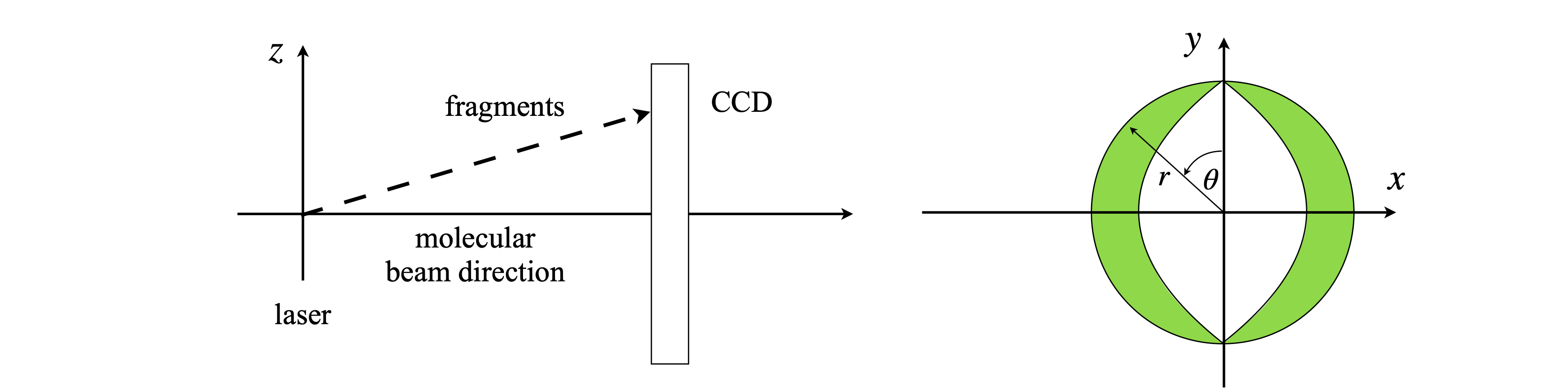# Questions 1 - 3#

## Q1 Graphite lattice#

In a teaching lab experiment to measure the lattice spacing of graphite, the angle at which the electrons diffract is measured as their accelerating voltage $$V$$ is changed. By the de-Broglie relationship, the electron’s wavelength is $$\lambda = h/p$$ where $$p$$ is the momentum. The relationship between kinetic energy and accelerating potential is $$eV = p^2/(2m)$$, hence, $$p = 2meV$$ where $$e$$ is the charge on the electron and $$m$$ its mass. Combining the wavelength and Bragg’s law $$n\lambda = 2d\sin(\theta)$$, produces the equation for the (first-order diffraction) inter-planar spacing $$d$$,

$\displaystyle d=\frac{h}{\sin(\theta)\sqrt{8meV}}$

In the experiment, the angle $$\theta$$ and the voltage are measured; all the other terms are constants. If the error on the angle is $$\sigma_\theta$$ and on the voltage $$\sigma_V$$V, what is the variance on $$d$$? Express the result in terms of $$d^2$$.

Strategy: Use equation 20, and differentiate in terms of $$\theta$$ and $$V$$. Simplify and substitute for $$d$$.

## Q2 Electrode potential#

The electrode potential $$E$$ of a reaction $$A_{ox} + ne^- \to A^-_{red}$$ is measured to obtain the ratio of activities of oxidized to reduced species, $$Q = a_{red} /a_{ox}$$ via the Nernst equation,

$\displaystyle E=E^\ominus -\frac{RT}{nF}\ln\left(\frac{a_{red}}{a_{ox}}\right)$

(a) If the standard deviation of the measured potential is $$\sigma_E$$ and $$\sigma_T$$ on the temperature, calculate how these affect the measured ratio of activities. Simplify the answer.

(b) At a given temperature, where will the standard deviation be smallest?

## Q3 Molecular beam#

Barlow (1989) gives an example of errors in a tracking chamber measured in cylindrical polar coordinates, and how they are related to errors in $$x, y$$, and $$z$$. This question examines a similar situation. In a molecular beam experiment, molecules are photo-dissociated and the image of the fragments, ions, or electrons is observed on a CCD camera at angle $$\theta$$ and distance $$r$$ from the origin at the centre of the detector, figure 6. This is also in the $$x-y$$ plane. There is an error in both $$\theta$$ and $$r$$ because the laser source has a certain size, and dissociation occurs from a finite volume in space, and because the molecules are themselves moving.

Calculate the error in $$x$$ and $$y$$ on the surface of the CCD camera.

The cylindrical polar coordinates have to be converted to Cartesians and the conversions are $$x = r\cos(\theta), y = r\sin(\theta)$$, and $$z = z$$. As the distance to the detector is large and fixed, assume that there is no error in $$z$$, and ignore this in the calculation.Figure 6. Geometry of a molecular beam photo-dissociation experiment and a sketch of an image as it might appear on a CCD camera. The oval shape is a consequence of the polarization of the absorption and rate of dissociation.

Strategy: Write down the $$G$$ matrix, which is also the Jacobian transformation between Cartesian and polar coordinates (see Chapter 4.11), and multiply out the matrices.